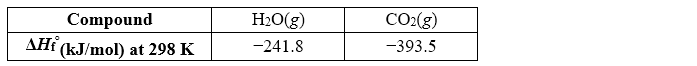# Problem: The explosive nitroglycerin (C3H5N3O9) decomposes rapidly upon ignition or sudden impact according to the following balanced equation: 4C3H5N3O9(l) → 12CO2(g) + 10H2O(g) + 6N2(g) + O2(g)    ΔH∘rxn =  −5678 kJ Calculate the standard enthalpy of formation (ΔH∘f) for nitroglycerin. A) −365.5 kJ B) −1462 kJ C) 1462 kJ D) −5678 kJ E) −5408.2 kJ

🤓 Based on our data, we think this question is relevant for Professor Tang's class at USF.

###### Problem Details

The explosive nitroglycerin (C3H5N3O9) decomposes rapidly upon ignition or sudden impact according to the following balanced equation:

4C3H5N3O9(l) → 12CO2(g) + 10H2O(g) + 6N2(g) + O2(g)    ΔHrxn =  −5678 kJ

Calculate the standard enthalpy of formation (ΔHf) for nitroglycerin.

A) −365.5 kJ

B) −1462 kJ

C) 1462 kJ

D) −5678 kJ

E) −5408.2 kJWhat scientific concept do you need to know in order to solve this problem?

Our tutors have indicated that to solve this problem you will need to apply the Enthalpy of Formation concept. You can view video lessons to learn Enthalpy of Formation. Or if you need more Enthalpy of Formation practice, you can also practice Enthalpy of Formation practice problems.

What is the difficulty of this problem?

Our tutors rated the difficulty ofThe explosive nitroglycerin (C3H5N3O9) decomposes rapidly up...as high difficulty.

How long does this problem take to solve?

Our expert Chemistry tutor, Dasha took 5 minutes and 1 second to solve this problem. You can follow their steps in the video explanation above.

What professor is this problem relevant for?

Based on our data, we think this problem is relevant for Professor Tang's class at USF.